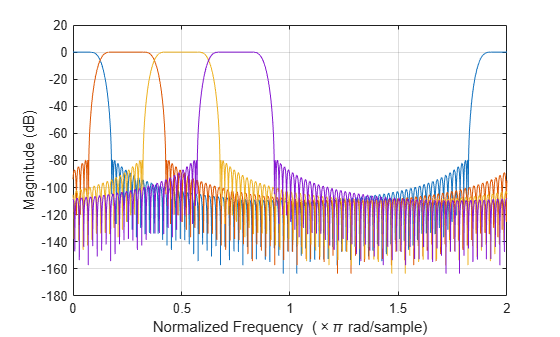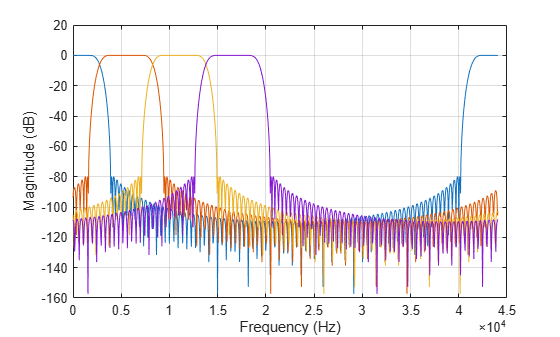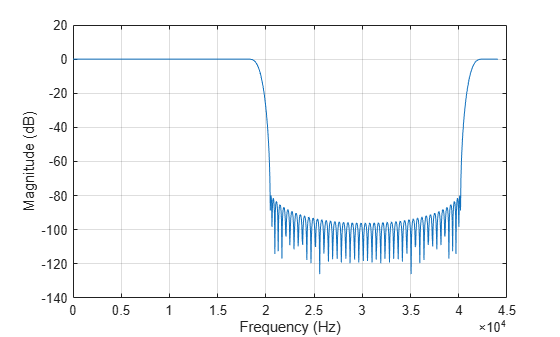# fvtool

Visualize the filters in the channelizer

## Syntax

``fvtool(obj)``
``fvtool(obj,ind)``
``fvtool(obj,ind,Name,Value)``

## Description

example

````fvtool(obj)` visualizes the filters in the `dsp.Channelizer` System object™ using the Filter Visualization Tool (FVTool).```

example

````fvtool(obj,ind)` visualizes the filters corresponding to the indices in the vector `ind`. `ind` is a row vector of indices between `1` and `obj.NumFrequencyBands`. By default, this vector is [1:N], where N is the smallest of `obj.NumFrequencyBands` and `64`.For example, to visualize the first 4 filters, set `ind` to `[1:4]`.channelizer = dsp.Channelizer; fvtool(channelizer,[1:4]);```

example

````fvtool(obj,ind,Name,Value)` visualizes the filters with additional options specified by one or more `Name,Value` pair arguments.For example, to visualize the first 4 filters in the channelizer over the Nyquist interval [–`44100/2`, `44100/2`] Hz, set `'Fs'` to `44100`. To compute the frequency response using 1024 frequency points, set `'NFFT'` to `1024`. In addition, to visualize sum of the filter responses, set `'overall'` to `true`.channelizer = dsp.Channelizer; fvtool(channelizer,[1:4],'Fs',44100,'NFFT',1024,'overall',true);```

## Examples

collapse all

Using the `fvtool` function, you can visualize the individual filter responses or sum of all the filter responses in the channelizer filter bank.

Design a channelizer with the number of frequency bands or polyphase branches set to 8, the number of taps or coefficients per band set to 12, and stopband attenuation set to 80 dB. View the response of the filter bank.

```channelizer = dsp.Channelizer; fvtool(channelizer)```The `fvtool` shows the response of the lowpass prototype filter and all the modulated filters. To view only a portion of the filter bank, specify the indices in `ind`. To view the response of the first 4 filters, set `ind` to [1:4].

`fvtool(channelizer,(1:4));`You can change the Nyquist interval to [-22,050 22,050] Hz and the number of frequency points to 1024.

`fvtool(channelizer,(1:4),'Fs',44100,'NFFT',1024);`To see the sum of the responses of all 4 filters, set the `'overall'` argument to `true`.

`fvtool(channelizer,(1:4),'Fs',44100,'NFFT',1024,'overall',true);`## Input Arguments

collapse all

Input filter, specified as a `dsp.Channelizer` System object.

Example: channelizer = dsp.Channelizer; fvtool(channelizer);

Filter indices, specified as a row vector in the range [1 `obj.NumFrequencyBands`]. By default, `ind` is set to 1:N, where N is the smallest of `obj.NumFrequencyBands` and `64`.

Example: fvtool(channelizer,[1:4]);

Data Types: `single` | `double` | `int8` | `int16` | `int32` | `int64` | `uint8` | `uint16` | `uint32` | `uint64`

### Name-Value Pair Arguments

Specify optional comma-separated pairs of `Name,Value` arguments. `Name` is the argument name and `Value` is the corresponding value. `Name` must appear inside quotes. You can specify several name and value pair arguments in any order as `Name1,Value1,...,NameN,ValueN`.

Example: fvtool(channelizer,'Fs',44100,'NFFT',1024,'overall',true);

Sampling rate, specified as a scalar. This value determines the Nyquist interval [-Fs/2 Fs/2] in which the fvtool shows the frequency response of the filters in the channelizer.

Example: 44100

Example: 22050

Data Types: `single` | `double` | `int8` | `int16` | `int32` | `int64` | `uint8` | `uint16` | `uint32` | `uint64`

Number of frequency points used to compute the frequency response, specified as a positive scalar.

Example: 8192

Example: 1024

Data Types: `single` | `double` | `int8` | `int16` | `int32` | `int64` | `uint8` | `uint16` | `uint32` | `uint64`

Type of filter response, specified as either:

• `true` –– `fvtool` shows the sum of the filter responses.

• `false` –– `fvtool` shows the individual filter responses.

Data Types: `logical`

Introduced in R2017b

Watch now